# 8.9 自定义图例

``````import matplotlib.pyplot as plt
plt.style.use('classic')

%matplotlib inline
import numpy as np

x = np.linspace(0, 10, 1000)
fig, ax = plt.subplots()
ax.plot(x, np.sin(x), '-b', label='Sine')
ax.plot(x, np.cos(x), '--r', label='Cosine')
ax.axis('equal')
leg = ax.legend();
``````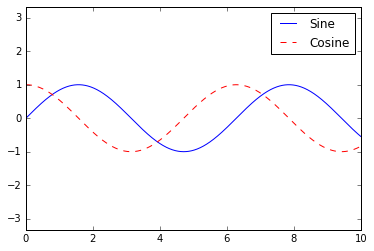``````ax.legend(loc='upper left', frameon=False)
fig
``````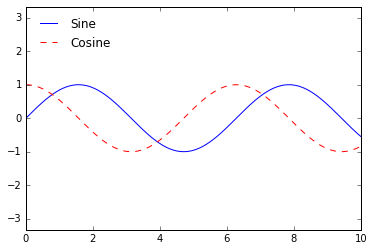``````ax.legend(frameon=False, loc='lower center', ncol=2)
fig
``````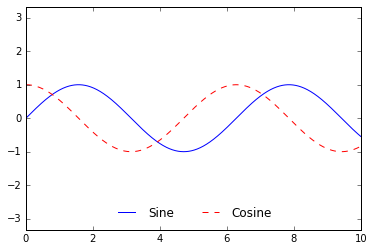``````ax.legend(fancybox=True, framealpha=1, shadow=True, borderpad=1)
fig
``````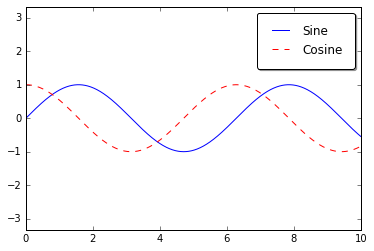## 为图例选择元素

``````y = np.sin(x[:, np.newaxis] + np.pi * np.arange(0, 2, 0.5))
lines = plt.plot(x, y)

# 直线是 plt.Line2D 实例的列表
plt.legend(lines[:2], ['first', 'second']);
````````````plt.plot(x, y[:, 0], label='first')
plt.plot(x, y[:, 1], label='second')
plt.plot(x, y[:, 2:])
plt.legend(framealpha=1, frameon=True);
``````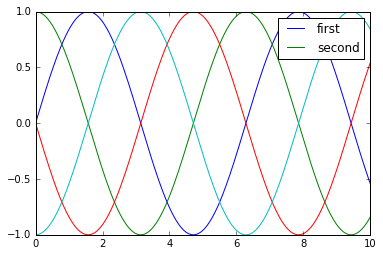## 用于点的大小的图例

``````import pandas as pd

# 提取我们感兴趣的数据
lat, lon = cities['latd'], cities['longd']
population, area = cities['population_total'], cities['area_total_km2']

# 将点绘制为散点图，使用尺寸和颜色，但没有标签
plt.scatter(lon, lat, label=None,
c=np.log10(population), cmap='viridis',
s=area, linewidth=0, alpha=0.5)
plt.axis(aspect='equal')
plt.xlabel('longitude')
plt.ylabel('latitude')
plt.colorbar(label='log\$_{10}\$(population)')
plt.clim(3, 7)

# 这里我们创建图例
# 我们使用所需大小和标签绘制空列表
for area in [100, 300, 500]:
plt.scatter([], [], c='k', alpha=0.3, s=area,
label=str(area) + ' km\$^2\$')
plt.legend(scatterpoints=1, frameon=False, labelspacing=1, title='City Area')

plt.title('California Cities: Area and Population');
``````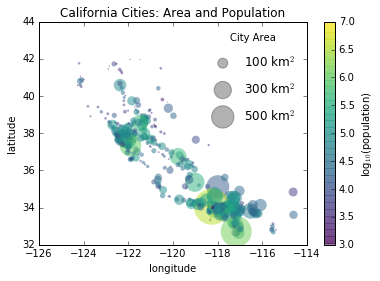## 多个图例

``````fig, ax = plt.subplots()

lines = []
styles = ['-', '--', '-.', ':']
x = np.linspace(0, 10, 1000)

for i in range(4):
lines += ax.plot(x, np.sin(x - i * np.pi / 2),
styles[i], color='black')
ax.axis('equal')

# 指定第一个图例的直线和标签
ax.legend(lines[:2], ['line A', 'line B'],
loc='upper right', frameon=False)

# 创建第二个图例，并手动添加艺术家
from matplotlib.legend import Legend
leg = Legend(ax, lines[2:], ['line C', 'line D'],
loc='lower right', frameon=False)